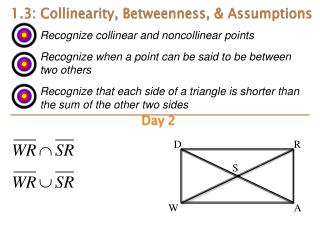DownloadDownload Presentation1.3: Collinearity , Betweenness , & Assumptions

# 1.3: Collinearity , Betweenness , & Assumptions

Download Presentation## 1.3: Collinearity , Betweenness , & Assumptions

- - - - - - - - - - - - - - - - - - - - - - - - - - - E N D - - - - - - - - - - - - - - - - - - - - - - - - - - -
##### Presentation Transcript

1. Recognize collinear and noncollinear points • Recognize when a point can be said to be between two others • Recognize that each side of a triangle is shorter than the sum of the other two sides 1.3: Collinearity, Betweenness, & Assumptions Day2 D R S W A

2. Collinearity • Collinear points are points that lie on the same line • Non-collinear points are points that do not lie on the same line Are A,B, and C collinear? CollinearNoncollinear C B A A C B

3. Betweenness Betweenness occurs when one point is between two others, but… …all three points must be collinear Is S between R and T? Yes No, not collinear S R S T R T

4. Triangle Inequality Any three points can be: • Collinear -One point between others • Noncollinear -Points determine a triangle

5. Triangle Inequality • If we are given three segment lengths we can determine if they are collinear or make up a triangle. • If 2 measures add up to the third then they make up a line • If the sum of 2 measures is greater than the third then they make up a triangle

6. Example 1 • Determine if the following three segments make up a line or a triangle. • AB=17, BC=24, AC=39 • Add up AB and BC AB+BC=17+24=41 • Compare AB+BC to AC AB+BC>AC • Since the sum of two of the sides is greater than the third side the three sides make up a triangle.

7. Assumptions from diagrams What can’t we assume? • Right angles • Congruent angles • Congruent segments • Relative size of segments or angles What can we assume? • Straight lines and angles • Collinearity • Betweenness • Relative positions

8. Example 2 Can assume D B E C A Cannot assume

9. Example 3 • Find M 5x x A I L

10. Example 3 Solution • Find M 5x x A I L

11. Example 4 If NO = 18, OT = 12, and NT = 30, which point is between the other two?

12. Example 4 Solution If NO = 18, OT = 12, and NT = 30, which point is between the other two? NT is the biggest segment so N and T are the endpoints. This means O is between the other two

13. Example 5 • What are the restrictions on EN? P 12 8 E N

14. Example 5 Solution If PN is the biggest side… PE + EN > PN 8 + EN > 12 EN > 4 So, 4 < EN < 20 If EN is the biggest side… PE+PN > EN 8 + 12 > EN 20 > EN